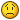# Calculate fastest side to rotate

## Recommended Posts

Hello guys!

Probably more a math problem than a phaser one, but still I'm breaking my head finding a solution. Maybe you can help me!

Imagine I have a sprite pointing towards the direction of the red arrow. I want to make the sprite rotate slowly towards the pointer (in this image the green arrow), and to have it rotate clockwise/counter-clockwise depending on which way is faster (in this case counter-clockwise).How can I calculate if the faster way is clock/counter-clockwise, providing the sprite can be pointing wherever and the pointer could be anywhere? If I was not clear I can try to clarify better##### Share on other sites

http://en.wikipedia.org/wiki/Cross_product

In computational geometry of the plane, the cross product is used to determine the sign of the acute angle defined by three points [...]##### Share on other sites

Ugh, I need to review my math!

Thanks for the quick reply, though unfortunately I am still unable to get the results I need. Maybe again some math noobism...

`function rotationSide(object, pointer){	oldAngle = object.rotation;	//Convert the angle to radians ranging from 0 to 2*PI	if (oldAngle < 0){		oldAngle = Math.abs(oldAngle);	}else{		oldAngle = 2*Math.PI - oldAngle;	}	x1 = object.x;	y1 = object.y;	x2 = object.x + Math.cos(oldAngle);	y2 = object.y + Math.sin(oldAngle);	x3 = pointer.worldX;	y3 = pointer.worldY;	return (x2-x1)*(y3-y1)-(y2-y1)*(x3-x1);}`

As defined by http://en.wikipedia.org/wiki/Cross_product#Computational_geometry, "the sign oftells whetherlies to the left or to the right of line", or in this case, the sign of the return should tell me if the pointer is to the left or to the right of the direction my sprite is pointing. But it is not doing that at the momentThanks!

##### Share on other sites

`function lerp_dir( cur_dir:Number , tar_dir:Number , inc:Number){		if ( Math.abs( tar_dir - cur_dir) <= inc or Math.abs( tar_dir - cur_dir) >= (360 - inc))	{		cur_dir = tar_dir;	}	else	{		if ( Math.abs( tar_dir - cur_dir) > 180)		{			if (tar_dir < cur_dir)			{				tar_dir += 360;			}			else			{				tar_dir -= 360;			}		}		if ( tar_dir > cur_dir)		{			cur_dir += inc;		}		else		{			if ( tar_dir < cur_dir)			{				cur_dir -= inc;			}		}	}	return cur_dir;}`

This was what I used a long time ago. Can't guarantee anything.

##### Share on other sites

Hello!

That code worked perfectly. I used sprite.rotation as cur_dir, angleToPointer(sprite) as tar_dir and a number like 0.05 as inc (increment). Also I changed 180 and 360 to Math.PI and 2*Math.PI to make it work with radiansThanks sbat and Fricken Hamster for the quick replies!

##### Share on other sites

I wrote that ages ago for as2, glad it worked.

##### Share on other sites

Posting this from my phone but this may work:

##### Share on other sites

• 1 year later...

Thank you very much for your solution Fricken Hamster.

After replacing the "or" statement and removing ":Number"s I managed to get it to work perfectly.

I have been trying to figure this out for so damn long..

##### Share on other sites

• 1 year later...

Hey there,

i used Fricken Hamster's Code in CreateJS, like this :

``````    function rotateDirection( current_angle, target_angle, increment){
if ( Math.abs( target_angle - current_angle) <= increment || Math.abs( target_angle - current_angle) >= (360 - increment))	{
current_angle = target_angle;
}
else
{
if ( Math.abs( target_angle - current_angle) > 180)		{
if (target_angle < current_angle)			{
target_angle += 360;
}
else
{
target_angle -= 360;
}
}
if ( target_angle > current_angle)		{
current_angle += increment;
}
else
{
if ( target_angle < current_angle)			{
current_angle -= increment;
}
}
}
return current_angle;
}``````

I don't know why, and exactly when it happens but there is a small problem. I have an Circle Shape that rotates it's face into mouseposition. When i turn my mouse several times around

the circle shape then SOMETIMES it doesn't turn incrementaly, but jumps directly about 180 degrees or somewhat. That does not look cool at all.

The function seems okay for me, so does anyone know what the problem is? I could make a small JSFiddle if that would be better for understanding my problem,

but the problem is, it does not happen always....it's on a specific degree when i turn the mouse and it jumps directly to it. I guess it has something to do with the first line

where current_angle is set equal to the target_angle....but why does it make so big jumps? the IF question only comes if the subtraction is smaller increment?

Did anyone else notice my problem, when using the code?

Greetings Mischka